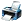If u^(5x)=v^(5y)=w^(5z) and u^2= vw then uv+vw-2wu=?

139 views

Multiple Choice Question
If u^(5x)=v^(5y)=w^(5z) and u^2= vw then uv+vw-2wu=?

Ans
1) 0
2) 1
3) 2
4) Noneposted May 30, 2018